【从0到0.1】数字签名随性笔记

# 0. 前言

## 0.1. MAC是什么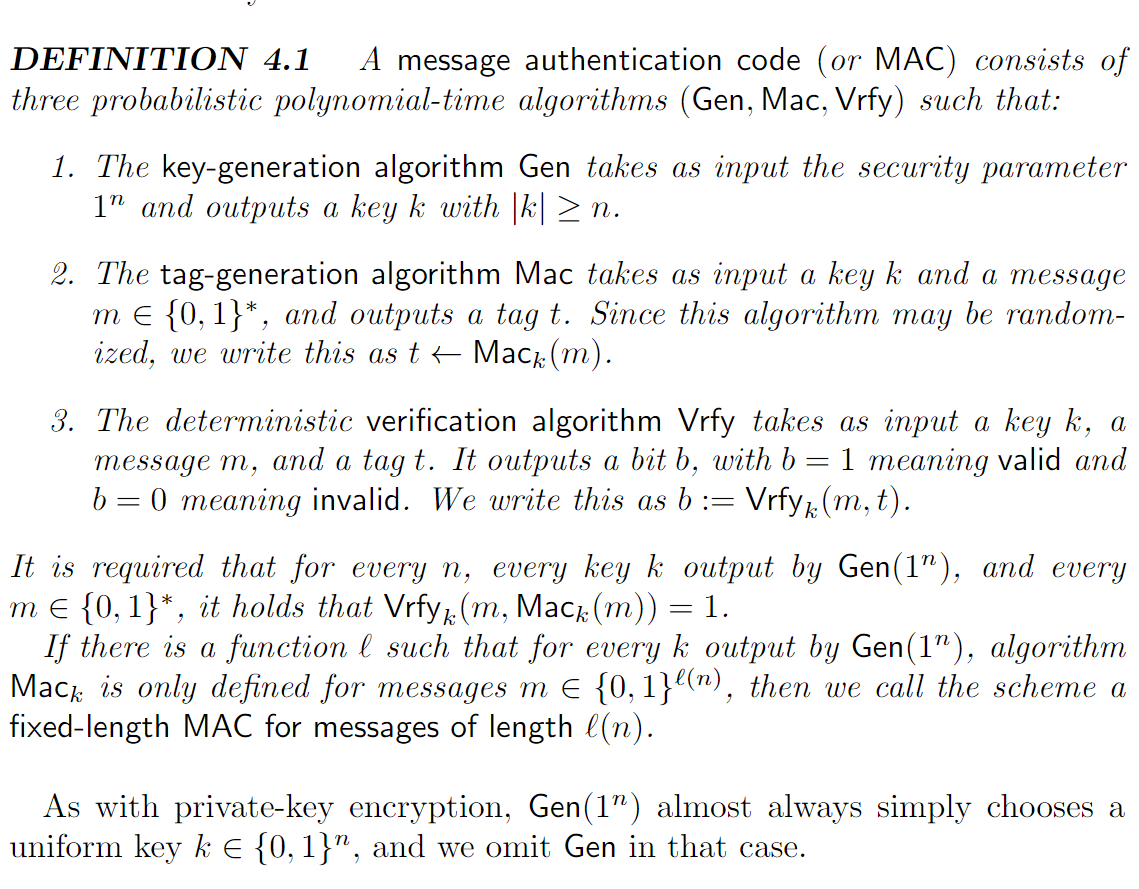## 0.2. 为什么需要MAC？

HTTP协议是无状态协议，无状态意味着：协议对于交互性场景没有记忆能力。

TBC

## 0.3. MAC的缺点是什么？/为什么需要数字签名？

MAC的一个非常显著的特点就是对称。Alice和Bob共享key，都可以构造tag并对(message,tag)进行验证——key的存在使得恶意第三方伪造正确的 (message,tag) 变得困难，但key的共享却带给了 Alice或Bob 抵赖的可能性。基于公钥密码思想的数字签名则解决了这个问题，但这不可避免地带来更多的开销，因此，在通信时若不要求：公开可验证、信任传递、不可抵赖性等特性的话，我们应当采用MAC来保证数据的完整性，而不是数字签名（考虑安全目标和敌手能力，再决定安全方案）。

# 1. 数字签名 安全定义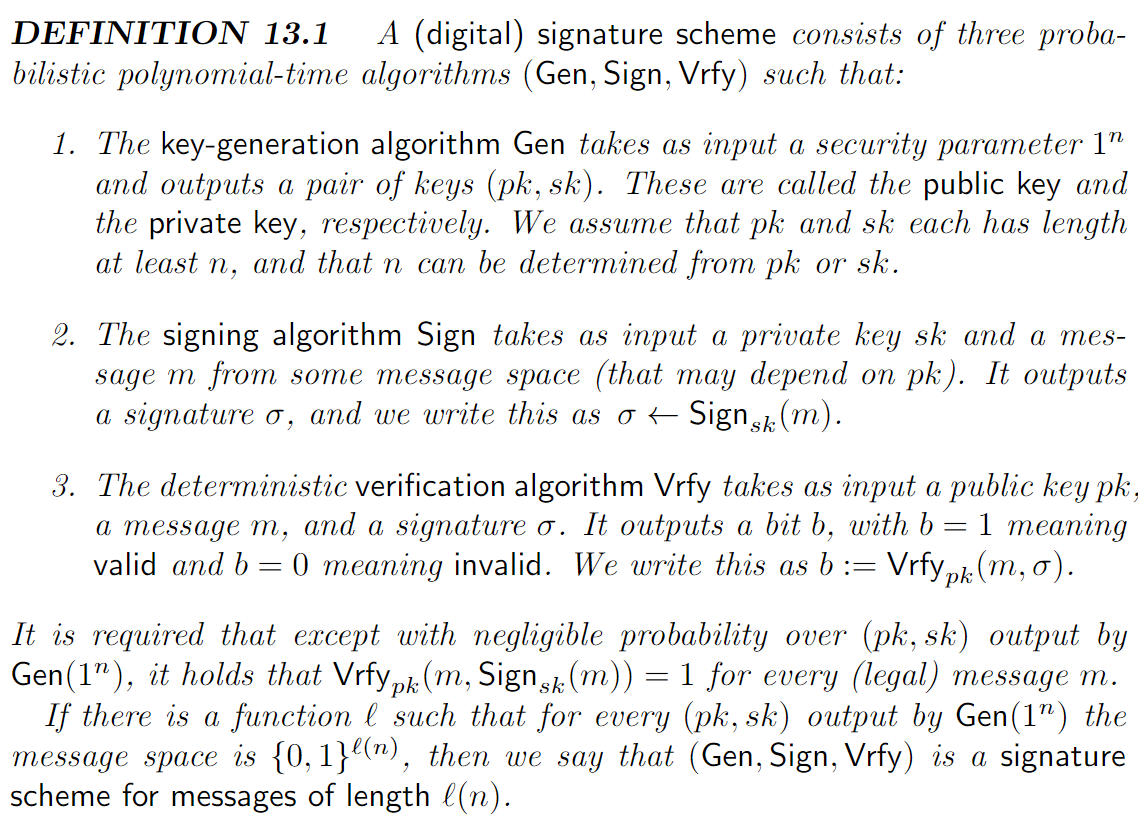# 2. RSA-Based Signatures

## 2.1. Plain RSA Signatures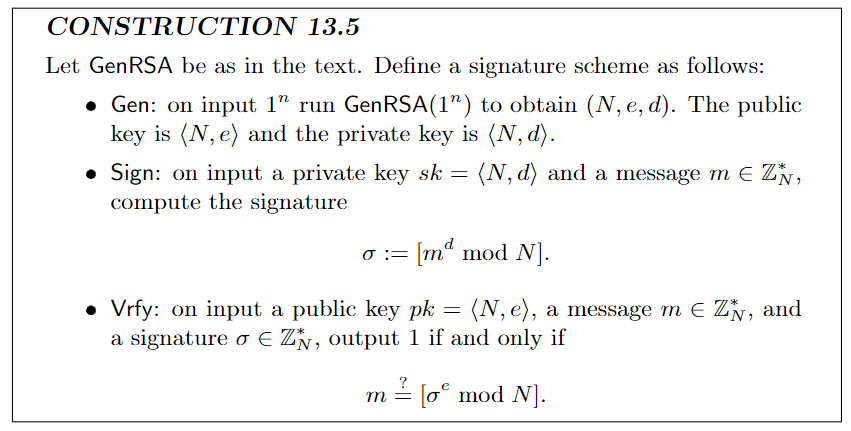1.No-message attack:

2.arbitrary message forgery:

## 2.2. RSA-FDH

RSA full-domain hash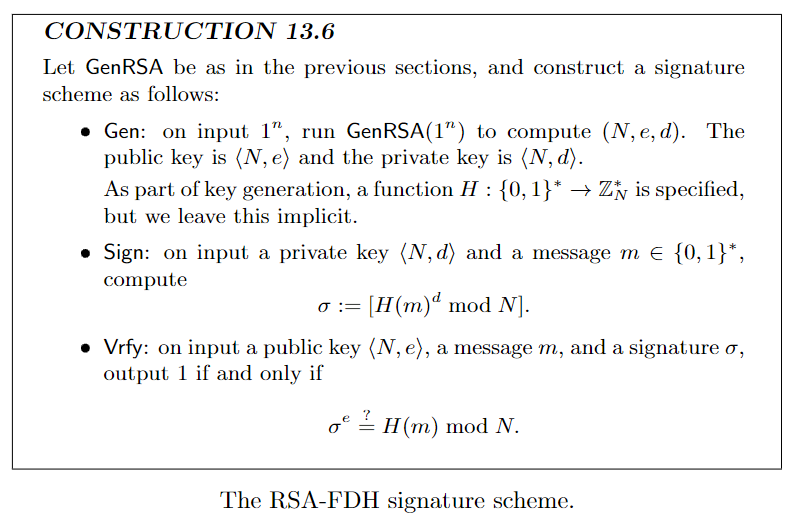# 3. DLP-Based Sig

## 3.1. Schnorr Sig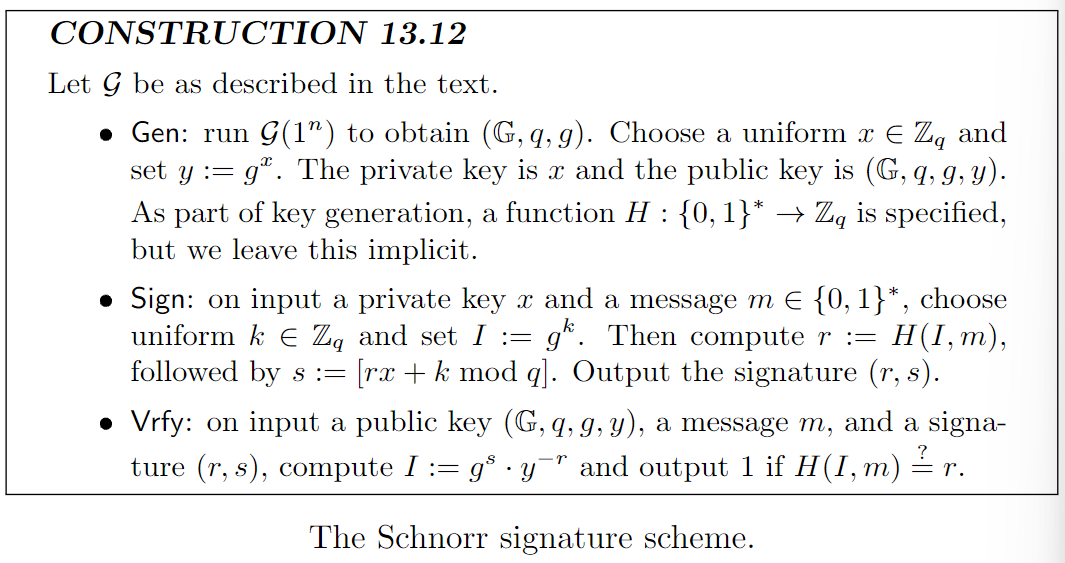Gen（密钥生成）：

（这三个是全局公钥参数,下文的是用户公\私钥参数）

Alice均匀随机抽取 $x \in \mathbb{Z}_q$ , 构造 $y = g^x\ mod\ p$ ，则有

$pk = (\mathbb{G},q,g,y)$

$sk = x$

Sign（签名）：

Alice均匀随机抽取 $k \in \mathbb{Z}_q$，构造 $I = g^k\ mod\ p$。该过程与m无关，可以预处理。

Vrfy（验签）：

Bob此时拥有 $pk，(m,(r,s))$

$g^sy^{-r} = g^{rx+k}g^{-rx} = g^k\ mod\ p$

## 3.2. DSA and ECDSA

DSA:Digital Signature Algorithm

ECDSA:Elliptic Curve Digital Signature Algorithm

DSA和ECDSA的抽象表述：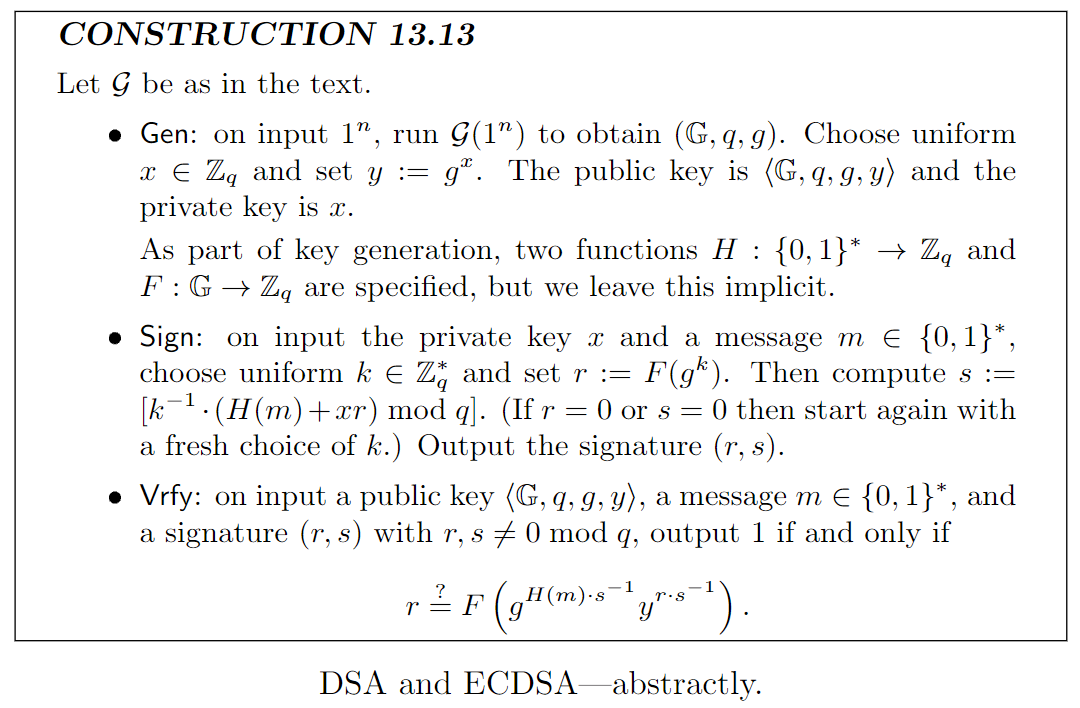### 3.2.1. DSA

DSA的公开参数选择与Schnorr签名方案完全一致：

Gen（密钥生成）：

（这三个是全局公钥参数,下文的是用户公\私钥参数）

Alice均匀随机抽取 $x \in \mathbb{Z}_q$ , 构造 $y = g^x\ mod\ p$ ，则有

$pk = (\mathbb{G},q,g,y)$

$sk = x$

Sign（签名）：

Alice均匀随机抽取 $k \in \mathbb{Z}_q$

Vrfy（验签）：

Bob此时拥有 $pk，(M',(r',s'))$

$u_1 = [H(M')w]\ mod\ q$

$u_2 = (r')w\ mod\ q$

$v = [(g^{u1})y^{u2}\ mod\ p]\ mod\ q$

TBC

### 3.2.2. ECDSA

ECDSA的全局域参数（全局公钥）：

Gen（密钥生成）：

Alice均匀随机抽取 $d \in \mathbb{Z}_n$ , 构造 $Q = dG$ ，则有

$pk = (E_q(a,b),G,n,Q)$

$sk = d$

Sign（签名）：

Alice均匀随机抽取 $k \in \mathbb{Z}_q$

Vrfy（验签）：

Bob此时拥有 $pk，(m,(r,s))$

$u_1 = H(m)w$

$u_2 = rw$

$X = u_1G+u_2Q = (x_1,y_1)$

TBC

# 4. 数字证书与PKI

PKI:公钥基础设施。

Charlie持有密钥对 $(pk_C,sk_C)$ ,与此同时Bob持有密钥对$(pk_B,sk_B)$。如果Charlie对于Bob的公钥是$pk_B$这一事实非常有把握，那么Charlie就可以为Bob与他的pk颁发数字证书：

$cert_{C \rightarrow B} = Sign_{sk_C}(Bob's\ key\ is\ pk_B)$

## 4.1. CA

### 4.1.3. Certificate chains

$cert_{B \rightarrow A} = Sign_{sk_B}(Alice's\ key\ is\ pk_A)$

$pk_A , cert_{B \rightarrow A} , pk_B , cert_{C \rightarrow B}$

Dave可以从中得到什么信息?

### 4.1.4. “web of trust” model

Alice 知悉三个CA $(C_1,C_2,C_3)$ 的三把公钥$pk_1,pk_2,pk_3$

Bob想要和Alice通信，他持有证书 $cert_{C_1 \rightarrow B}$, $cert_{C_3 \rightarrow B}$ , $cert_{C_4 \rightarrow B}$ ，他会把这些证书连同他的公钥$pk_B$ 发送给Alice

# 4.2. 无效证书

## 4.2.1. Expiration

Expiration:终结

$cert_{C \rightarrow B} = Sign_{sk_C}(Bob's\ key\ is\ pk_B,date)$

## 4.2.2. Revocation

Revocation:撤销

$cert_{C \rightarrow B} = Sign_{sk_C}(Bob's\ key\ is\ pk_B,\#\#\#)$

###代表每个证书特有的序列号

CA维护一个 certificate revocation list (CRL，证书吊销列表)，其中包含了每个被撤销的证书的序列号。CA每隔一段时间都会更新一次并为它签名。

# 5.TLS

TLS:Transport Layer Security (protocol)

TLS 允许 client (e.g.,browser) 与 server (e.g.,website) 就一组共享密钥达成一致，然后使用这些密钥进行加密与验证后续通信。它包含两部分：负责密钥交换（和认证）的握手协议和使用共享密钥加密\认证的记录层协议。

## 5.1. 握手协议

server S 持有（用来签名的）公私钥对$(pk_S,sk_S)$以及数字证书$cert_{i \rightarrow S}$，用以绑定 $pk_S$ 与 S。

1. C与S进行 DHE (Diffie-Hellman Ephemeral) 密钥交换，包括公共参数$(\mathbb{G},q,g)$ (可能是$Z_p^*$ , 或一个椭圆曲线群……)， 以及 一个随机数 $x$ 构造$g^x$ , 同时C会通知对方自己支持哪些加密算法

2. S与C进行 DHE 密钥交换，构造$g^y$。然后，S可以计算 $K = g^{xy}$ 。再通过密钥派生函数(key derivation function)，利用 $K$ 派生密钥 $k_S',k_C',k_S,k_C$ , 用在认证加密(authenticated encryption,AE)方案中。

最后，S发送 $pk_S$ 和证书 $cert_{i \rightarrow S}$ , 以及 一份数字签名 $\sigma$ (用长期密钥$sk_S$签名从开端至今所有交换的握手消息)。这些值用 $k_S'$ 加密。

3. C 计算 K , 以相同的方法派生密钥 $k_S',k_C',k_S,k_C$ , 使用 $k_S'$ 解密上述密文恢复 $pk_S$、数字证书与 $\sigma$ 。C 验证数字证书，从而相信 $pk_S$ 是 S 的公钥，然后再验证 $\sigma$ 。最后，C利用 $k_C'$ 计算握手消息的MAC 并发给S。在进入记录层协议之前，S验证该tag。

### 5.1.1 为什么握手协议是安全的？

TLS 1.2 版本允许 C 和 S 通过公钥加密方式交换密钥（而不是DH 密钥交换），在这种情况下，S持有的密钥对$(pk_S,sk_S)$ 就是用作公钥加密。此时C简单地选取K并用$pk_S$ 加密（该协议的细节略有不同，特别是C在验证$pk_S$的正确性之前便已完成加密过程）

DHE则不会带来这方面的问题。注意到E指的是Ephemeral（短暂的），一旦握手完成，服务器中的随机秘密值y便可以立刻被擦除，没有了y，敌手便无从获取K。

TLS1.3 版本不允许通过公钥加密的方式交换密钥以保证前向安全。

## 5.2. 记录层协议

C用 $k_C$ 认证加密 信息

S用 $k_S$ 认证加密 信息

（双方不用同一个key是为了抵抗反弹攻击）

# 参考

Introduction to Modern Cryptography